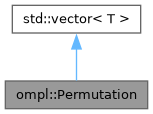ompl::Permutation Class Reference

A permutation of indices into an array. More...

#include <ompl/datastructures/Permutation.h>

Inheritance diagram for ompl::Permutation:[legend]

## Public Member Functions

Permutation (std::size_t n)
Create a permutation of the numbers 0, ... , n - 1.

void permute (unsigned int n)
Create a permutation of the numbers 0, ..., n - 1.

## Detailed Description

A permutation of indices into an array.

This class tends to be faster than the two-argument version of std::random_shuffle when permute is called several times, since the random number generator doesn't need to be allocated each time.

Definition at line 81 of file Permutation.h.

The documentation for this class was generated from the following file: